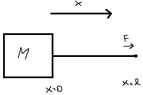# Block of mass M attached to rope of mass m

AspiringPhysicist12
Homework Statement:
A uniform rope of mass m and length l is attached to a block of
mass M. The rope is pulled with force F. Find the tension at distance
x from the end of the rope. Neglect gravity.
Relevant Equations:
T(0) = T(L) = Ma
We have the string's mass constraint m(x) = m(x/L). The block is accelerated right by force T(x=0) = Ma, where a = F / m + M.

But at the point where the force is applied (i.e. x = L), I believe we have m(L/L)a = ma = F - T(L). But this would imply T(L) = F - ma = F - m(F / m + M) = F(M / m + M) = Ma. I know it doesn't make sense to have T(0) = T(L) since the rope has mass, but what am I doing wrong here?

#### Attachments

•1653505282845.png
711 bytes · Views: 36

Homework Helper
Gold Member
2022 Award
Homework Statement:: A uniform rope of mass m and length l is attached to a block of
mass M. The rope is pulled with force F. Find the tension at distance
x from the end of the rope. Neglect gravity.
Relevant Equations:: T(0) = T(L) = Ma

We have the string's mass constraint m(x) = m(x/L). The block is accelerated right by force T(x=0) = Ma, where a = F / m + M.

But at the point where the force is applied (i.e. x = L), I believe we have m(L/L)a = ma = F - T(L). But this would imply T(L) = F - ma = F - m(F / m + M) = F(M / m + M) = Ma. I know it doesn't make sense to have T(0) = T(L) since the rope has mass, but what am I doing wrong here?
I can't follow what you are doing. Why not start with the tension at ##x = 0##?

Staff Emeritus
Homework Helper
Gold Member
I believe we have m(L/L)a = ma = F - T(L)
Why?

AspiringPhysicist12
Sorry let me reword it a bit. If I use the constraint T(0) = Ma, then that gives me a final answer of T(x) = F(m(x/L) + M)/(m + M). This would mean T(0) = Ma as expected, but also that T(L) = F. I don't understand why T(L) = F.

Gold Member
Homework Statement:: A uniform rope of mass m and length l is attached to a block of
mass M. The rope is pulled with force F. Find the tension at distance
x from the end of the rope. Neglect gravity.
Relevant Equations:: T(0) = T(L) = Ma

We have the string's mass constraint m(x) = m(x/L). The block is accelerated right by force T(x=0) = Ma, where a = F / m + M.

But at the point where the force is applied (i.e. x = L), I believe we have m(L/L)a = ma = F - T(L). But this would imply T(L) = F - ma = F - m(F / m + M) = F(M / m + M) = Ma. I know it doesn't make sense to have T(0) = T(L) since the rope has mass, but what am I doing wrong here?
I believe you want to start by presenting FBD's of the block and an arbitrary length of rope ## x ##, and one of the remaining length of rope.

Homework Helper
Gold Member
2022 Award
Sorry let me reword it a bit. If I use the constraint T(0) = Ma, then that gives me a final answer of T(x) = F(m(x/L) + M)/(m + M). This would mean T(0) = Ma as expected, but also that T(L) = F. I don't understand why T(L) = F.
What do you think it should be?

Hint: consider that the end of the rope has a small handle of small mass ##m_0##. Find the tension in the rope attached to the handle. Now take ##m_0 = 0##.

•AspiringPhysicist12
AspiringPhysicist12
Right, it makes sense in that case but that would mean the mass at the end of the rope is 0? Why do we take the rope's mass as zero there and as the entire mass m where the rope and block meet.

Homework Helper
Gold Member
2022 Award
Right, it makes sense in that case but that would mean the mass at the end of the rope is 0? Why do we take the rope's mass as zero there and as the entire mass m where the rope and block meet.
It was just to give you an idea on why the tension must be ##F## at the end of the rope where there is no more mass.

Gold Member
Right, it makes sense in that case but that would mean the mass at the end of the rope is 0? Why do we take the rope's mass as zero there and as the entire mass m where the rope and block meet.
I think at the "end" of the rope the tension must be ## F ## because it is accelerating the mass ## M ## and all of the mass of the rope ## m_r ## at ##a##. Near the block, the tension force is a reaction to accelerating the mass ## M ## at ##a##, and thus will be less than ##F##

Does that explain it, or have I not understood it properly myself?

•AspiringPhysicist12